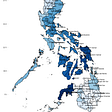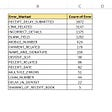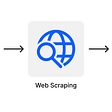# Visualize Gapminder Data in R: A Step-by-Step Tutorial

## Learn to visualize the impact of real-world events

In case you have taken an introductory statistics/data science course, you must have noticed that instructors often use idealized datasets — with rows and columns in the perfect shape. However, as we step into real-world data analysis, it does not take long for us to realize that such datasets are just fictitious creations (of course with the noble purpose of easing our entry into the complex world of data).

After finishing some introductory courses, I was looking for some real-world datasets which are fun to work with but, at the same time, contain some challenges to deal with. And I could not have found a better source than Gapminder! I am quoting their goal directly from their website: “Gapminder fights devastating misconceptions and promotes a fact-based worldview everyone can understand.” In case you are not yet familiar with Gapminder, here is the link to their website: https://www.gapminder.org/data/

In this article, using multiple packages in R, I will try to explain how to visualize (also, how to tell a story) with the data freely available on the gapminder website.

As an example, we will try to visualize how the per capita GDP one of the key indicators of economic growthof India and China evolved over the last 70 years.

Step 2: Load the data in R

Next, I create a folder in which both my R code (.R file) and the downloaded data named gdppercapita_us_inflation_adjusted are located. Then, in R, I click Session → Set Working Directory → To Source File Location. And we are all set to start coding!

`#Load the data and name it g (or whatever you wish to name it!)g<-read.csv("gdppercapita_us_inflation_adjusted.csv")`

Also, load all the required packages:

`library(dplyr)library(ggplot2)library(tidyr)`

Now, let’s have a quick look at the dataframe g just to determine our next steps:

Looking at the dataframe, I decide to do the following:

1. Change the name of the first column (not sure why it got messed up like this). We will name it Country. This step is not necessary though!
`#Changing the name of the first columncolnames(g)[colnames(g) == "ï..country"] <- "Country"`

Okay, so we learned how to change a column name in R!

2. The dataframe is presented in wide format (which means it has more columns than rows). Ideally, we want it in a long format (which means it should have more rows than columns). We do that by creating a new column named Year; and for each Country and Year, we want the value of per capita GDP to be under the Per_Capita_GDP column. I use the pivot_longer () function from the tidyr package for converting the data from wide to long format.

`#Wider to Longer Formatg2 <- g %>%  pivot_longer(    cols = starts_with("X"),    names_to = "Year",    names_prefix = "X",    values_to = "Per_Capita_GDP",    values_drop_na = TRUE  )`

And, we learned how to change the shape of a dataframe in R!

3. Next, I want to change the K to thousand (1000).

`#Changing the k to thousand (1000)g2\$Per_Capita_GDP<-as.numeric(sub("k", "e3", g2\$Per_Capita_GDP, fixed = TRUE))`

Cool! Our dataframe is ready for visualization!

Step 3: Select India and China

`#Selecting India and China [filter () function from the dplyr package]g3<-g2%>% filter(Country=='India'|Country=='China')`

Step 4: Create a Line Chart

`ggplot(data=g3, aes(x=Year, y=Per_Capita_GDP, group=Country,color=Country)) +  geom_line(size=2.5)`

Well, it worked but we have a lot to improve! Let’s fix things one by one:

1. This dataframe contains data from 1959 to 2019. We do not want to show all these years on the x-axis as we do not have enough space. Rather, let’s mention only specific years.

(From now on, I write the extra codes, on top of the existing code, in bold)

`ggplot(data=g3, aes(x=Year, y=Per_Capita_GDP, group=Country,color=Country)) + geom_line(size=2.5)+scale_x_discrete(breaks=c(1960,1970,1980,1990,2000,2010,2019))`

2. We should add a title to the graph and add labels to the x and y axes.

`ggplot(data=g3, aes(x=Year, y=Per_Capita_GDP, group=Country,color=Country)) +geom_line(size=2.5)+scale_x_discrete(breaks=c(1960,1970,1980,1990,2000,2010,2019))+ xlab("Year")+ylab("GDP/Capita, PPP\$ Inflation-Adjusted")+ggtitle("Comparing Per Capita GDP of India and China over the Years")`

3. Let’s bring the title to the middle (by typing hjust=0.5 inside the theme function). Also, we can change the font sizes of the title and the axes labels. Additionally, we can change the panel background from the default gray to white and have only horizontal grid lines.

`ggplot(data=g3, aes(x=Year, y=Per_Capita_GDP, group=Country,color=Country)) +geom_line(size=2.5)+scale_x_discrete(breaks=c(1960,1970,1980,1990,2000,2010,2019))+  xlab("Year")+ylab("GDP/Capita, PPP\$ Inflation-Adjusted")+ggtitle("Comparing Per Capita GDP of India and China over the Years")+  theme(plot.title    = element_text(face = "bold",   size = 25, hjust = 0.5), axis.text.x   = element_text(size=15),        axis.text.y   = element_text(size=15),        axis.title.x  = element_text(size=15),        axis.title.y  = element_text(size=15),        legend.text   = element_text(size=15),        legend.title  = element_text(size=15),        panel.background = element_rect(fill = "white"),        panel.grid.major.y = element_line(colour = "grey10"))`

4. If we want to change the colors of the lines:

`ggplot(data=g3, aes(x=Year, y=Per_Capita_GDP, group=Country,color=Country)) +geom_line(size=2.5)+scale_x_discrete(breaks=c(1960,1970,1980,1990,2000,2010,2019))+  xlab("Year")+ylab("GDP/Capita, PPP\$ Inflation-Adjusted")+ggtitle("Comparing Per Capita GDP of India and China over the Years")+  theme(plot.title    = element_text(face = "bold",   size = 25, hjust = 0.5),        axis.text.x   = element_text(size=15),        axis.text.y   = element_text(size=15),        axis.title.x  = element_text(size=15),        axis.title.y  = element_text(size=15),        legend.text   = element_text(size=15),        legend.title  = element_text(size=15),        panel.background = element_rect(fill = "white"),        panel.grid.major.y = element_line(colour = "grey10")) +   scale_color_manual(values=c("#CC6666", "#9999CC"))`

Okay! Now, let’s try to understand the story hidden in the data. It seems like from 1959 to 1975, the per capita GDP of both countries was following an almost parallel trend; however, something happened in China close to 1980 and their economy has been booming ever since!

After quick research on this, here is what I found: it appears that the stunning economic success of China was led by their visionary leader Deng Xiaoping, who rose to preeminence in 1977.

Back to our graph! Let’s finish it off by adding a vertical line on 1977 and mentioning the historic event of Xiaoping’s rise to power:

`ggplot(data=g3, aes(x=Year, y=Per_Capita_GDP, group=Country,color=Country)) +  geom_line(size=2.5)+scale_x_discrete(breaks=c(1960,1970,1980,1990,2000,2010,2019))+  xlab("Year")+ylab("GDP/Capita, PPP\$ Inflation-Adjusted")+ggtitle("Comparing Per Capita GDP of India and China over the Years")+  theme(plot.title    = element_text(face = "bold",   size = 25, hjust = 0.5),        axis.text.x   = element_text(size=15),        axis.text.y   = element_text(size=15),        axis.title.x  = element_text(size=15),        axis.title.y  = element_text(size=15),        legend.text   = element_text(size=15),        legend.title  = element_text(size=15),        panel.background = element_rect(fill = "white"),        panel.grid.major.y = element_line(colour = "grey10")) +         scale_color_manual(values=c("#CC6666", "#9999CC"))+  geom_vline(xintercept = 19, linetype="dashed", color = "red", size=1.5)+  annotate(geom="text", x=35, y=5000, label="Deng Xiaoping's Rise to Preeminance", color="red",size=6)`

Obviously, there are more things that we can do with this data. But, for now, we end right here.

I hope this article piqued your curiosity to explore, analyze, and visualize more real-world events with data!

--

--

--

## More from Vivekananda Das

Sharing ideas on Cause-and-Effect, Data Analysis in R, Stat Literacy, and Happiness | Ph.D. student @UW-Madison | More: https://vivekanandadas.com

Love podcasts or audiobooks? Learn on the go with our new app.

## Let’s Paint the Town Red! — Visualizing the Election Data Results## 7 Step Strategy to Build Data Products and Platforms## Equilibrium & The APT Theory## Dare to Be Different in the Data Science Community## 1. How to make sense of 270k clinical trial descriptions## How to create a Pareto Chart in Excel — 80/20 Rule or Pareto Principle## How does location affect the price of Airbnb in Prague## The best tools for Dashboarding in Python## Vivekananda Das

Sharing ideas on Cause-and-Effect, Data Analysis in R, Stat Literacy, and Happiness | Ph.D. student @UW-Madison | More: https://vivekanandadas.com

## Web Scraping in R with Rvest## Why you should use Swarmplots for Data Visualization## The Data Sandbox | Speed cameras in Toronto## EP-Edina, MN Home values in 2022: Exploratory Data Analysis (EDA) in R programming .